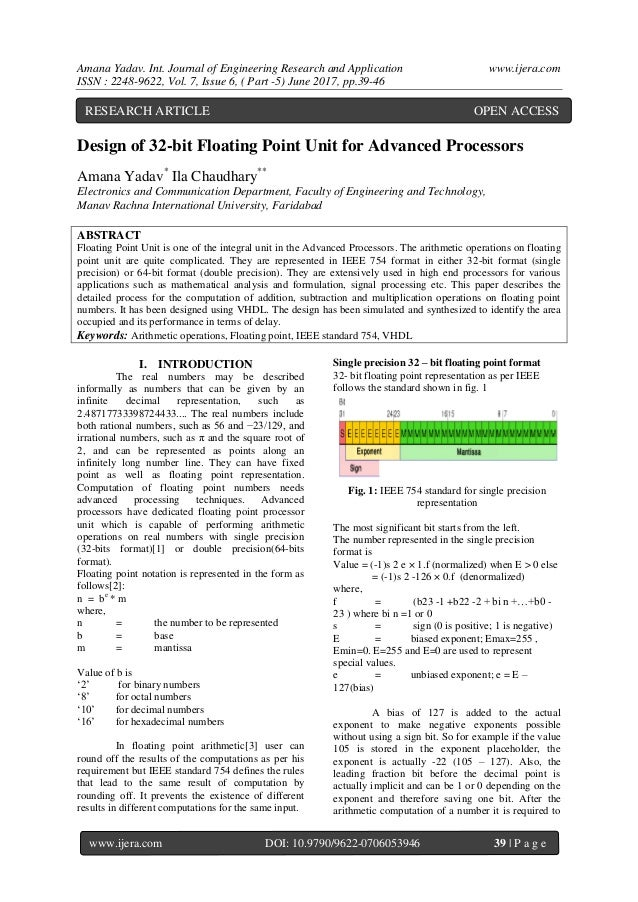Successfully reported this slideshow.Upcoming SlideShare
×

# Design of 32-bit Floating Point Unit for Advanced Processors

41 views

Published on

Floating Point Unit is one of the integral unit in the Advanced Processors. The arithmetic operations on floating point unit are quite complicated. They are represented in IEEE 754 format in either 32-bit format (single precision) or 64-bit format (double precision). They are extensively used in high end processors for various applications such as mathematical analysis and formulation, signal processing etc. This paper describes the detailed process for the computation of addition, subtraction and multiplication operations on floating point numbers. It has been designed using VHDL. The design has been simulated and synthesized to identify the area occupied and its performance in terms of delay.

Published in: Engineering
• Full Name
Comment goes here.

Are you sure you want to Yes No• Be the first to comment

• Be the first to like this